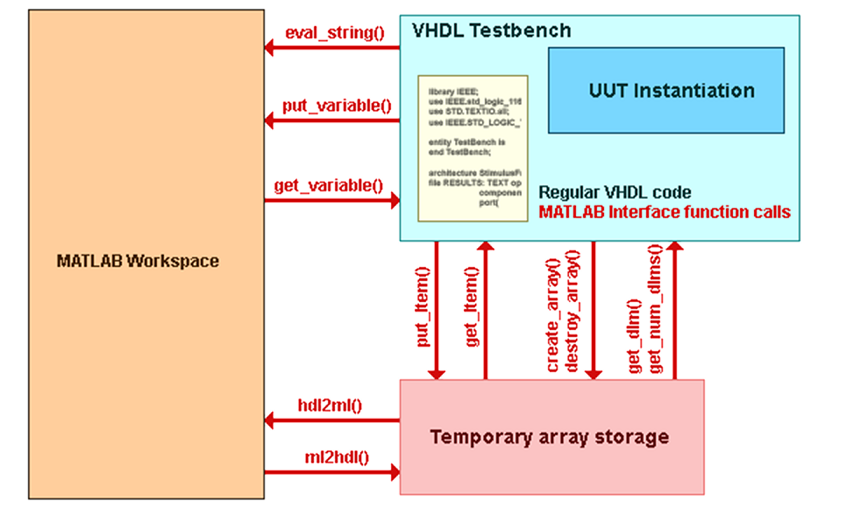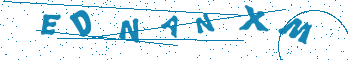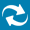# Matlab – HDL Interface in Riviera-PRO and Active-HDL

## Introduction

Active-HDL and Riviera-PRO provide a built-in interface that allows the integration of The MathWorks' intuitive language and a technical computing environment with Aldec's HDL-based simulation environment. The interface included in Active-HDL/Riviera-PRO allows executing MATLAB® commands, calling M-functions, or transfering data to or from the MATLAB workspace. All operations are controlled from your HDL code. Communication with MATLAB is accomplished with a dedicated set of subprograms prepared for both Verilog and VHDL. At any level of a design hierarchy, you can pass commands to MATLAB (e.g. pass an expression to solve or call M-function), transfer HDL variables to MATLAB workspace, perform necessary operations, and transfer the results back to the HDL simulator.

The interface consists of:

• The libaldec_matlab_cosim.so shared object library (Linux) or the aldec_matlab_cosim.dll dynamic-link library (Windows). The library contains a set of VHDL foreign subprograms and Verilog system tasks that allow to execute commands in the MATLAB environment and transfer data between the HDL simulator and the MATLAB workspace. The library is located in the bin/ subdirectory

• The VHDL matlab package compiled to the aldec library. The package contains foreign subprograms declarations.## For Linux - Configuring \$LD_LIBRARY_PATH Variable

Before you start using the Interface to MATLAB on Linux, the \$LD_LIBRARY_PATH system variable must be defined and it must contain paths to necessary MATLAB resources:

• \$matlabroot/bin/<platform>

• \$matlabroot/extern/lib/<platform>

Assuming that \$matlabroot points to /usr/local/matlab and the <platform> string equals glnx86 the following commands could be used to configure the environment:

For the bash shell:

LD_LIBRARY_PATH=/usr/local/matlab/bin/glnx86:/usr/local/matlab/extern/lib/glnx86:\$LD_LIBRARY_PATH

export LD_LIBRARY_PATH

For the csh shell:

setenv LD_LIBRARY_PATH

/usr/local/matlab/bin/glnx86:/usr/local/matlab/extern/lib/glnx86:\$LD_LIBRARY_PATH

## For Windows - Registering a COM Server

When you install MATLAB on your computer, it registers a COM server with the operating system. But in some cases you need to register a COM server before you start using the Interface to MATLAB on Windows manually. This can be done with the following command at the operating system command prompt:

matlab /regserver

The command should be entered once and you do not need to re-enter it after a system restart

## Enable Interface Operations for Verilog and VHDL

### For Verilog - Declaring PLI Library

MATLAB -related task and functions are located in the libaldec_matlab_cosim.so (Linux) or aldec_matlab_cosim.dll (Windows) library. This library must be added to the list of PLI applications visible to the simulator. Otherwise the simulator will not be able to find (and execute) those tasks and functions.

• Select the Preferences Dialog Box | Compilation | Verilog | Entries category or the Preferences Dialog Box | Simulation |Verilog | Entries category. The list of PLI applications is shared by the compiler and the simulator. Setting of the Compilation |Verilog | Entries category are not obligatory unlike the Simulation | Verilog | Entries category. Use the option Show entries for: PLI Applications to locate the libaldec_matlab_cosim.so (Linux) or aldec_matlab_cosim.dll(Windows) library.

• An equivalent of the PLI Applications option is setting the \$user_pli variable with the set command. This variable contains a list of PLI applications to be loaded by the simulator at simulation initialization.

• The required PLI application can also be specified with -pli argument for the asim command. See Verilog PLI and VPI for details.

### For VHDL - Declaring MATLAB Packages

In VHDL, the routines for interfacing MATLAB are located in package matlab compiled to the aldec library. The package must be declared in VHDL source code. To declare the package, do the following:

1. Use the following clause in VHDL source code:

library aldec;

use aldec.matlab.all;

2. Make sure that the aldec library is visible to the simulator and package matlab is compiled into it. This can be done with the following commands:

alist

## Interface Functions

### Controlling MATLAB and Configuring the Interface

Functions in this group can be used to send commands to MATLAB® and configure default values for arguments in functions responsible for data transfers between Active-HDL/Riviera-PRO and MATLAB. You can also specify whether MATLAB should bring up its desktop when it starts.

eval_string procedure call is used to execute a MATLAB “.m” file as shown by a snippet of VHDL code below. Note that a MATLAB “.m” file is simply a collection of MATLAB commands that may be entered at the MATLAB command prompt. So a simple command that changes the directory in MATLAB is used in eval_string too, i.e. any MATLAB command that is executed at the command prompt is permitted in the eval_string command as part of the HDL testbench, whether VERILOG or VHDL.

```MATLAB_INIT : process
variable dim_constr : TDims(1 to 2) := (1, 1024);
begin
eval_string("cd src");
eval_string("prepare_workspace");
```

Note: the file “prepare_workspace.m” exists in the source directory.

### Passing Scalar Values between Active-HDL/Riviera-PRO and MATLAB

The following routines are supported by the MATLAB® interface to pass and manipulate scalar values:

put_variable procedure call is used to send a VHDL constant, variable, or signal to MATLAB as shown by a snippet of VHDL code below.

```constant window_type : integer := 4;
signal in_wave : std_logic_vector (15 downto 0);
MATLAB_WINDOW : process (clk)
variable i : integer;
begin
put_variable("w", window_type);
put_variable("input", in_wave);
put_variable("index", i);
eval_string("windowed_input = input * windows1024(w).coeff(index);");
```

FROM MATLAB:

> w>

w =

4

Note: if “input”, “index”, or “windowed_input” is entered at the MATLAB command prompt, the value will be displayed as it was for “w”.

get_variable procedure call is used to assign a MATLAB value to a VHDL variable as shown by a snippet of VHDL code below.

```MATLAB_WINDOW : process (clk)
variable Qin_var : std_logic_vector(15 downto 0);
begin
eval_string("windowed_input = input * windows1024(w).coeff(index);");
get_variable("windowed_input", Qin_var);
```

Note: VHDL variable “Qin_var” from the MATLAB_WINDOW VHDL process is assigned the “windowed_input” MATLAB value.

put_simtime procedure call transfers the VHDL simulation time to a MATLAB value, and is executed from a VHDL process as shown by a snippet of VHDL code below.

```ANALYZE_FFT : process (clk)
variable dim_constr : TDims(1 to 2) := (1, 1);
begin
if rising_edge(clk) then
if start = '1' then
dim_constr(2) := 1;
end if;
if OE = '1' then
put_simtime("UPDATED_TIME");--VHDL simulation time is transferred to the “UPDATED_TIME” MATLAB value.
dim_constr(2) := dim_constr(2) + 1;
end if;
if (dim_constr(2) = 1025) then
dim_constr(2) := 1;
end if;
end if;
end process;
```

FROM MATLAB:

> UPDATED_TIME>

UPDATED_TIME =

0.1409

### Passing and Manipulating Array Values

The MATLAB interface allows passing and manipulating multi-dimensional arrays with HDL routines. For efficiency, the arrays are created in the interface space and not copied to the HDL simulator. Thus routines are used to create arrays first and then data are transferred.

The following routines are provided:

create_array function call is used to create a temporary storage array from a VHDL process as shown by a snippet of VHDL code below. The temporary storage array is given an identifier (Id) and is transferred/updated to MATLAB via the hdl2ml procedure call as shown below.

```---- Architecture declarations -----
shared variable Qin_Id : INTEGER := 0;
shared variable Iout_Id : INTEGER := 0;
shared variable Qout_Id : INTEGER := 0;

MATLAB_INIT : process
variable dim_constr : TDims(1 to 2) := (1, 1024);
begin
Qin_Id := create_array("fft_Qin", 2, dim_constr);   --fft_Qin is set to be a 2 dimensional array [1 X 1024] in temporary storage
Iout_Id := create_array("fft_Iout", 2, dim_constr); --fft_Iout is set to be a 2 dimensional array [1 X 1024] in temporary storage
Qout_Id := create_array("fft_Qout", 2, dim_constr); --fft_Qout is set to be a 2 dimensional array [1 X 1024] in temporary storage
hdl2ml(Iout_Id);   --fft_Iout is sent to MATLAB
hdl2ml(Qout_Id); --fft_Qout is sent to MATLAB
hdl2ml(Qin_Id); --fft_Qin is sent to MATLAB
```

FROM MATLAB:

> fft_Qin>

fft_Qin =

Columns 1 through 12

183 183 184 184 184 184 184 184 185 185 185 -186

Columns 13 through 24

...........

Columns 1021 through 1024

184 184 183 -184

destroy_array procedure call is used to remove a temporary storage array from a VHDL process as shown by a snippet of VHDL code below. The known temporary storage array identifier (Id) must be given as shown below.

```---- Architecture declarations -----
shared variable Qin_Id : INTEGER := 0;

MATLAB_INIT : process
variable dim_constr : TDims(1 to 2) := (1, 1024);
begin
Qin_Id := create_array("fft_Qin", 2, dim_constr);   --fft_Qin is set to be a 2 dimensional array [1 X 1024] in temporary storage
hdl2ml(Qin_Id); --fft_Qin is sent to MATLAB
destory_array(Qin_Id);  -- fft_Qin has been deleted/removed from temporary storage and therefore no updates possible to MATLAB,
--i.e. “fft_Qin” still remains in MATLAB but can’t be updated
```

put_item procedure call is used to store a VHDL constant, variable, or signal in a temporary storage array and is executed from a VHDL process as shown by a snippet of VHDL code below. The known temporary storage array identifier (Id) must be given as shown below. The pointer or reference element must be given in order to properly populate the matrix.

```---- Signal declarations ---
signal Qin : std_logic_vector (15 downto 0);

DISPLAY_INPUT : process (clk,reset)
variable dim_constr : TDims(1 to 2) := (1, 1);  -- (row,column) of an (M X N) matrix, in this example M = 1 and N = 1024
-- and begin populating matrix at [1,1], i.e. [row,col]
begin
if reset = ‘1’ then
dim_constr(2) := 1;
end if;
put_item(Qin, 0, Qin_Id, dim_constr);--the VHDL signal “Qin” pointed to as “Qin” as the starting point has
--each element assigned to the “fft_Qin” matrix
--as shown in the previous example.  The temporary storage “fft_Qin” 2 dimensional
--array reference element is given in the “dim_constr” value and starts at [1,1]
--and goes to [1,1024] in populating the matrix, so it is a row vector of 1024 elements.
dim_constr(2) := dim_constr(2) + 1;
if (dim_constr(2) = 1025) then
hdl2ml(Qin_Id);   --fft_Qin is sent to MATLAB, i.e. “fft_Qin” is updated so all previous values in MATLAB are replaced with new ones.
eval_string("display_input");   --file “display_input.m” exists in the source directory
dim_constr(2) := 1;
end if;
end process;
```

ml2hdl function call transfers an array from MATLAB to a temporary storage array and is executed from a VHDL process as shown by a snippet of VHDL code below. get_item procedure call is then used to obtain a given element of the array and store it in a VHDL variable.The known temporary storage array identifier (Id) is obtained from the ml2hdl procedure call within the VHDL process. The pointer or reference element must be given in order to obtain the correct value from the MATLAB matrix or array.

```---- Architecture declarations -----;
shared variable Iin_Id : INTEGER := 0;

ANALYZE_FFT : process (clk)
variable dim_constr : TDims(1 to 2) := (1, 1);-- (row,column) of an (M X N) matrix, in this example M = 1 and N = 1024
variable Iin_test : STD_LOGIC_VECTOR(15 downto 0); -- variable required to store element of array obtained from MATLAB
begin
if rising_edge(clk) then
if start = '1' then
dim_constr(2) := 1;
end if;
if OE = '1' then
Iin_Id := ml2hdl("fft_Iin");--fft_Iin is an array in MATLAB and that array is transferred to a temporary
-- storage space and is accessed using the Identifier Iin_Id
get_item(Iin_test,0,Iin_Id,dim_constr); -- the VHDL variable “Iin_test”  pointed to as “Iin” as the
-- starting point has its single value assigned to the “fft_Iin” matrix
-- element pointed to by “dim_constr”.  The temporary storage “fft_Iin”
-- 2 dimensional array reference element is given in
-- the “dim_constr” value and starts at [1,1] and goes to [1,1024] which
--	is the MATLAB matrix, i.e. a row vector of 1024 elements.
dim_constr(2) := dim_constr(2) + 1;
end if;
end if;
end process;
```

get_num_dims function call obtains the dimensions of a MATLAB matrix (array) in temporary storage space, i.e. if given an (M X N X K) it will return a value of 3 within the VHDL process. get_dim function call will return the value for K if passed a 3, M if passed a 1, and N if passed a 2. Both function calls are executed from a VHDL process as shown by a snippet of VHDL code below.

```---- Architecture declarations -----;
shared variable Iin_Id : INTEGER := 0;

ANALYZE_FFT : process (clk)
variable dim_constr : TDims(1 to 2) := (1, 1);-- (row,column) of an (M X N) matrix, in this example M = 1 and N = 1024
variable Iin_test : STD_LOGIC_VECTOR(15 downto 0); -- variable required to store element of array obtained from MATLAB
variable Iin_total_dimensions : INTEGER;
variable Iin_size_last_dimension : INTEGER;
begin
if rising_edge(clk) then
if start = '1' then
dim_constr(2) := 1;
end if;
if OE = '1' then
Iin_Id := ml2hdl("EKF");--EKF is an array in MATLAB and that array is transferred to a temporary storage
--space and is accessed using the Identifier Iin_Id
Iin_total_dimensions := get_num_dims(Iin_Id);--EKF is a [1 X 1024] matrix, so this procedure call will return the value of 2.
Iin_size_last_dimension := get_dim(Iin_Id,Iin_total_dimensions);--the procedure call will return the value of 1024.
dim_constr(2) := dim_constr(2) + 1;
get_item(Iin_test,0,Iin_Id,dim_constr);
end if;
if (dim_constr(2) =  Iin_size_last_dimension + 1) then
dim_constr(2) := 1;
end if;
end if;
end process;
```

get_time procedure call obtains the MATLAB simulation time and is executed from a VHDL process as shown by a snippet of VHDL code below.

```ANALYZE_FFT : process (clk)
variable dim_constr : TDims(1 to 2) := (1, 1);
begin
if rising_edge(clk) then
if start = '1' then
dim_constr(2) := 1;
end if;
if OE = '1' then
get_time;--Benchmark procedure
dim_constr(2) := dim_constr(2) + 1;
end if;
if (dim_constr(2) = 1025) then
dim_constr(2) := 1;
end if;
end if;
end process;
```

## Class and Cast Mnemonics

The class and cast mnemonics for VHDL are defined in the matlab package compiled to the aldec library. Class mnemonics are listed in the enumeration mxClassID type and cast mnemonics in the mxCastID enumeration type.

The class and cast mnemonics for VHDL are defined in the matlab package compiled to the aldec library. Class mnemonics are listed in the enumeration mxClassID type and cast mnemonics in the mxCastID enumeration type. When used in the Verilog source code, the mnemonic should be precede with the accent grave ( ` ) character.

## Example FFT Program

Both Active-HDL and Riviera-PRO come with “fft_analysis” sample design that demonstrate the usage of Matlab interface.

• Active-HDL: the default design location of the sample design is C:\My_Designs\Samples\matlab_fft_analysis\

• Riviera-PRO: the design is located in <Riviera-PRO_Install_Dir>/examples/tools/matlab. It is advised that you copy it to a separate directory where all user Riviera-Pro designs are kept.

In Active-HDL/Riviera Pro load the fft_analysis workspace file from the user directory. Compile the fft_analysis workspace and then execute the runme.do file (in Riviera-PRO comment out the cd command in runme.do file first), observe the resulting waveforms generated in MATLAB.

Open the VHDL test bench file “top_fft_tb.vhd” and notice how the eval_string, put_variable, create_array, get_variable, put_item, and hdl2ml commands are used.